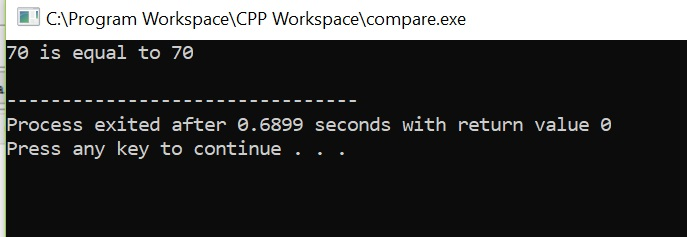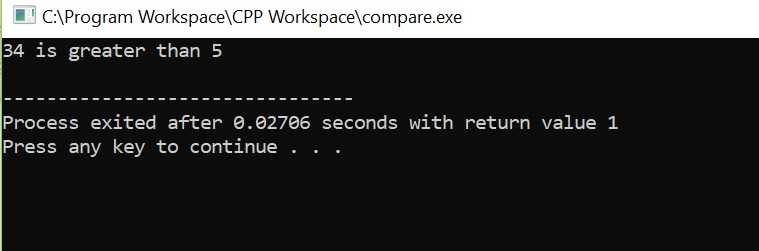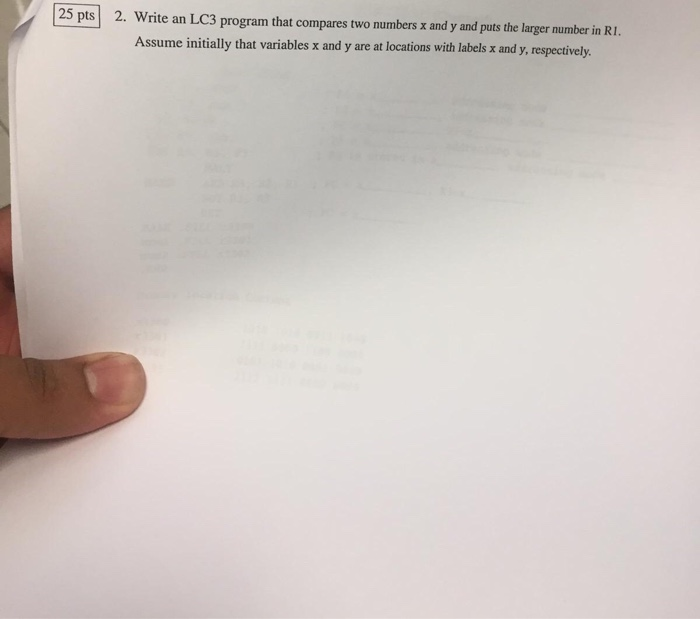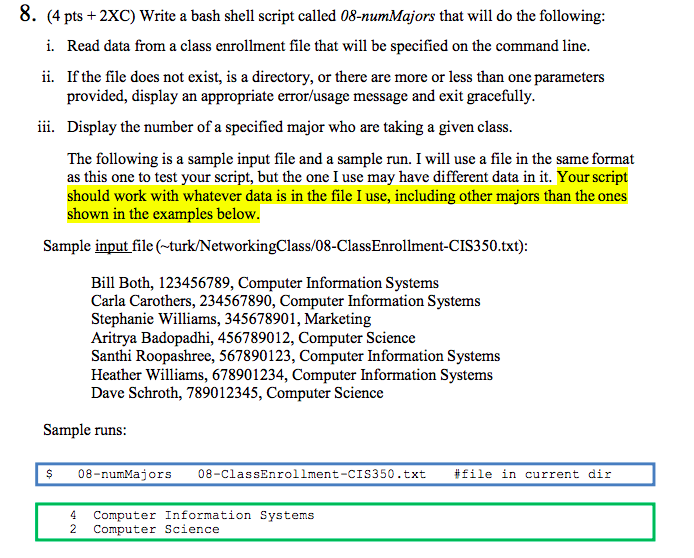# Write a program in C or a script in bash, called “compare” that takes two numbers on the command line and compares them....

Write a program in C or a script in bash, called “compare” that takes two numbers on the command line and compares them. The program should print the result of the comparison. Specifically, it should print “<x> is <comparison> <y>”, where <x> is the first number, <y> is the second number and <comparison> is one of “equal to”, “greater than” or “less than”. If the two numbers are equal, the program should have an exit status of zero. The exit status should be non-zero in all other cases. Use pseudo-code if necessary, but try to use valid C or bash syntax wherever possible. You may write the program and test it on UNIX. Write or copy/paste your code here.

 C Program ```#include int main(int numargs, char **argv) { // check if the number of command line arguments is 3 or not if(numargs == 3) { // convert the arguments to integers int x = atoi(argv); int y = atoi(argv); // compare the numbers if( x > y ) printf("%d is greater than %d\n", x, y ); else if(y > x) printf("%d is less than %d\n", x, y ); // else they are equal else { printf("%d is equal to %d\n", x, y ); return 0; } } // outer else else printf("Invalid number of arguments supplied!"); return 1; }```

OUTPUT##### Add Answer of: Write a program in C or a script in bash, called “compare” that takes two numbers on the command line and compares them....
Similar Homework Help Questions
• ### unix bash shell script

Create a BASH shell script called momoaccording to the following specifications:"# Your script will be used to organize a collection of MP3 files.# Files to be processed are expected to reside in a single directory# (no sub-directories).# Your script, must create subdirectories for each artist and# create symbolic links to each song in appropriate directories.# The script usage will be as follows:# momo source_directory [destination directory]# If the destination directory is not provided, the present working directory# is the destination.#...

• ### Write a program that asks the user to enter two numbers. The program should then print on the...

Write a program that asks the user to enter two numbers. The program should then print on the screen one of the following messages depending on the values of thetwo numbers:a) the first number is smaller than the second numberb) the first number is larger than the second numberc) the first number is equal to the second numberI'm not exactly sure how to do the c++ while statements. Thank you very much for the help!

• ### | 25 pts 25 pts) 2. 2. Write an LC3 program that compares two numbers x...| 25 pts 25 pts) 2. 2. Write an LC3 program that compares two numbers x and y and puts the larger number in RI. Assume initially that variables x and y are at locations with labels x and y, respectively.

• ### 8. (4 pts + 2Xc) Write a bash shell script called 08-numMajors that will do the following i. Read data from a class enrollment file that will be specified on the command line ii. If the file does no...8. (4 pts + 2Xc) Write a bash shell script called 08-numMajors that will do the following i. Read data from a class enrollment file that will be specified on the command line ii. If the file does not exist, is a directory, or there are more or less than one parameters provided, display an appropriate error/usage message and exit gracefully Display the number of a specified major who are taking a given class iii. The following is a sample...

• ### Write a C program countFiles.c to be executed on the command line as follows: countFiles <directory> The program...

Write a C program countFiles.c to be executed on the command line as follows: countFiles <directory> The program should count the (regular) files in the specified directory as well as all subdirectories and output the total number on the console. Files and subdirectories whose names .start with should be ignored! To do this, define a function int countFilesRec(char* dirName)that dirName returns the number of (regular) files in the directory and all the subdirectories. Call the function recursively to count the...

• ### FOR JAVA Write a program that takes two command line arguments: an input file and an...

FOR JAVA Write a program that takes two command line arguments: an input file and an output file. The program should read the input file and replace the last letter of each word with a * character and write the result to the output file. The program should maintain the input file's line separators. The program should catch all possible checked exceptions and display an informative message. Notes: This program can be written in a single main method Remember that...

• ### In Python: Write a program that determines whether two circles intersect. The input should consist of...

In Python: Write a program that determines whether two circles intersect. The input should consist of six numbers, x1, y1, r1 and x2, y2, r2, given as command-line arguments, and representing the center and radius of the two circles. Your program should print True if the two circles intersect, and False otherwise. As a reminder, the two circles intersect if and only if the distance of their two centers is less than or equal to the sum of their two...

• ### Write a program **(IN C)** that displays all the phone numbers in a file that match the area code...

Write a program **(IN C)** that displays all the phone numbers in a file that match the area code that the user is searching for. The program prompts the user to enter the phone number and the name of a file. The program writes the matching phone numbers to the output file. For example, Enter the file name: phone_numbers.txt Enter the area code: 813 Output: encoded words are written to file: 813_phone_numbers.txt The program reads the content of the file...

• ### Write a C program to run on ocelot to read a text file and print it...

Write a C program to run on ocelot to read a text file and print it to the display. It should optionally find the count of the number of words in the file, and/or find the number of occurrences of a substring, and/or take all the words in the string and sort them lexicographically (ASCII order). You must use getopt to parse the command line. There is no user input while this program is running. Usage: mywords [-cs] [-f substring]...

• ### using C , comments will be appreciated. Question1 Write down an function named bitwisedFloatCompare(float number1, float...

using C , comments will be appreciated. Question1 Write down an function named bitwisedFloatCompare(float number1, float number2)that tests whether a floating point number number1is less than, equal to or greater than another floating point number number2, by simply comparing their floating point representations bitwise from left to right, stopping as soon as the first differingbit is encountered. The fact that this can be done easily is the main motivation for biased exponent notation. The function should return 1 if number1...

Need Online Homework Help?